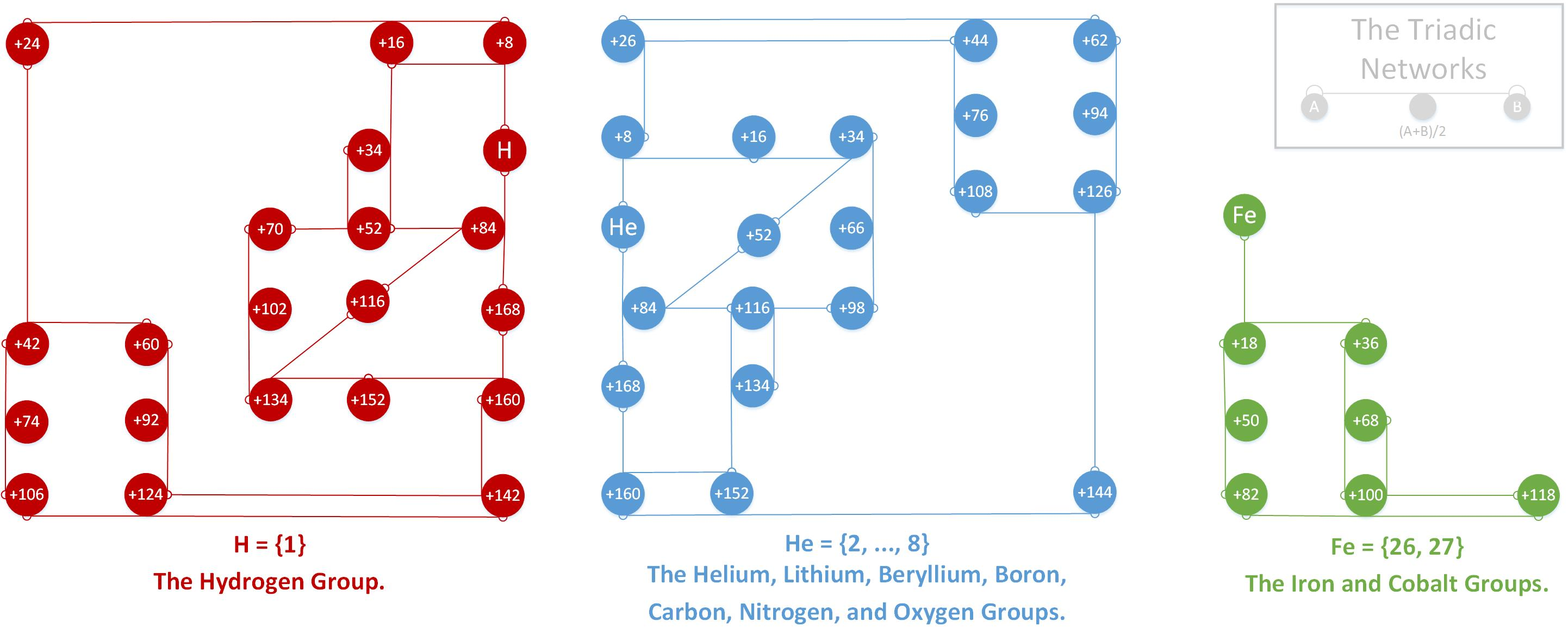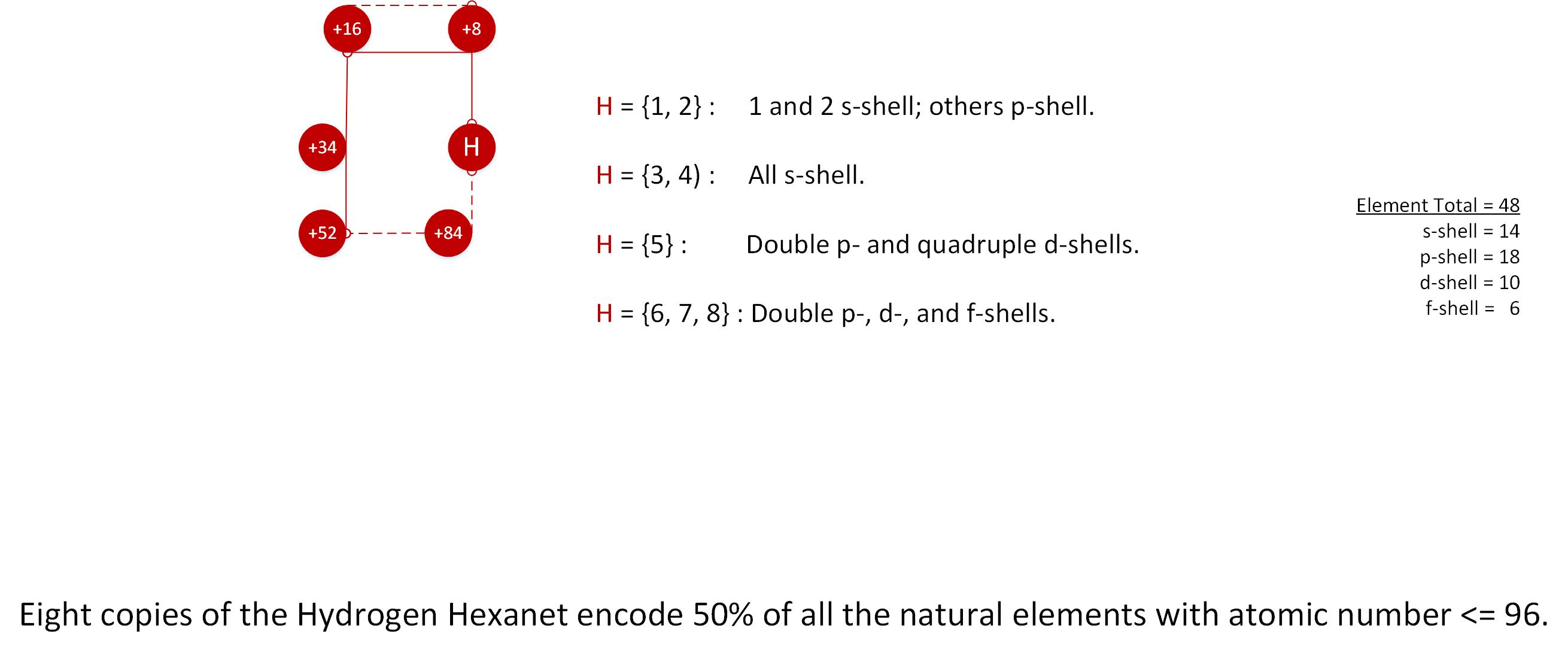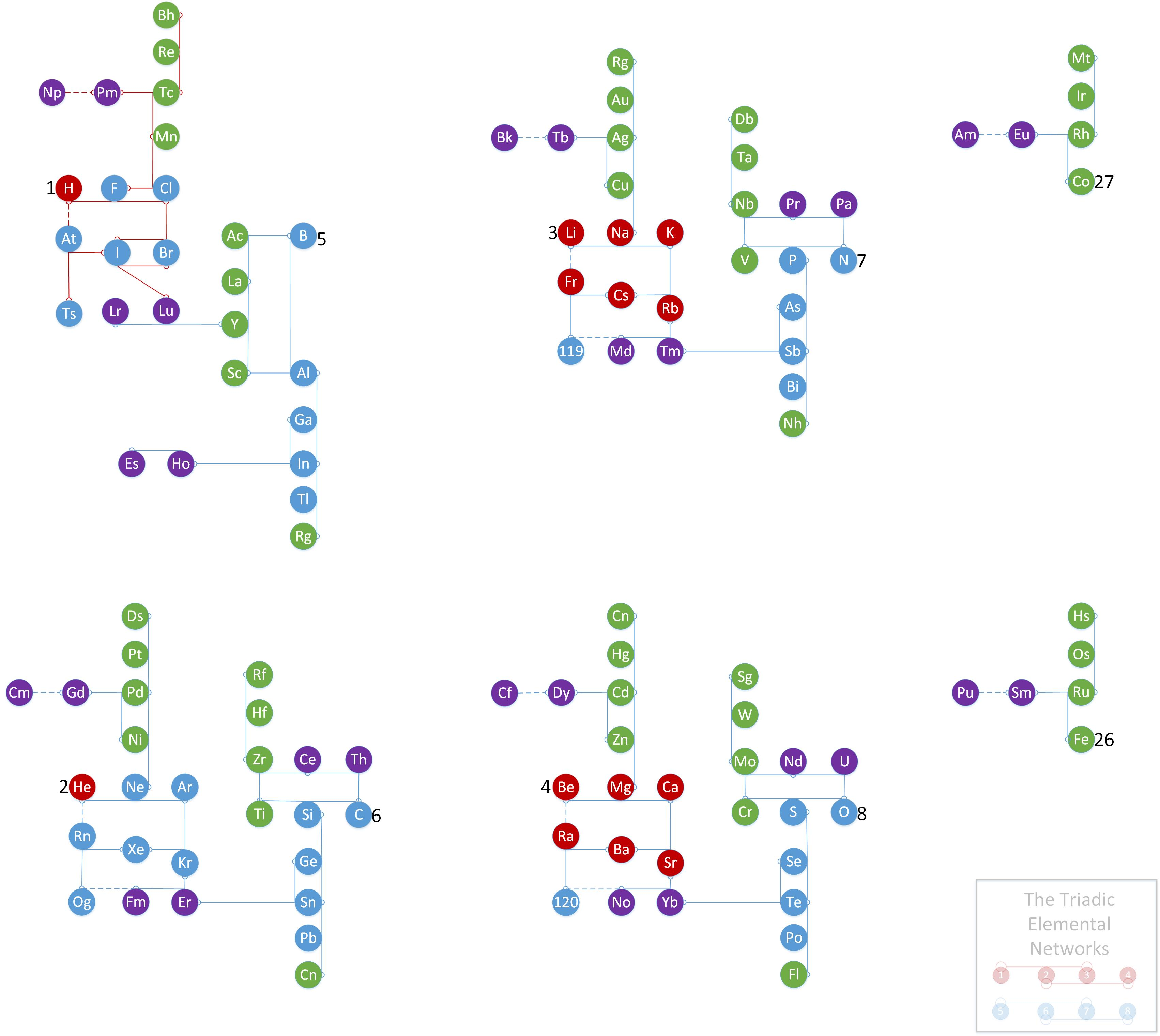# The Triadic Networks: periodicity for the 21st century.

This is a great summary paper by Dr. Eric Scerri on the role of triads in evolution of the periodic table.

This is the paper by Dr. Alfio Zambon which inspired this work.

Here then is my contribution to chemistry: the Triadic Networks (TN), which is a general mathematical design, and the Triadic Elemental Networks (TEN), which apply that design to chemical elements.

I will be filling in more as need be but for now I’m putting up the diagrams that are necessary and sufficient to understand the concept.

I look forward to discussing this further with you and everyone else!!This is a general math relationship based on the triadic average, where the middle numer of a link is the average of the outer two numbers.  There are many ways to do this in a set of numbers; here we see one such design inspired by Zambon’s Periodicity Tree 0 (Figure 3 in his paper).FIGURE 2: THE HYDROGEN HEXANET.
As the figure itself says, this draws attention to the fact that a 6-element collection of linked numbers (or elements) which we call a hexanet is responsible for half the elements that occur naturally on earth and, based on predictions, in the universe.FIGURE 3: THE TRIADIC ELEMENTAL NETWORKS.
Fully expanding figure 4 and linking redundancies generates this new version of the periodic table.  Here we see four layers of even and odd numbers connected by triads.  As the legend indicates, the layers are inter-connected by two linear triadic networks, 1234, and 5678, and layers 2, 3, and 4 are more strongly connected to each other than layer 1.